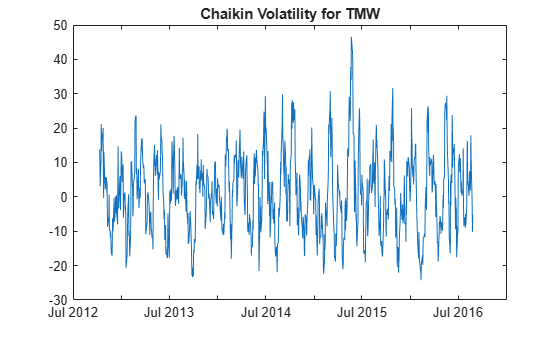Main Content

# chaikvolat

Chaikin volatility

Using a `fints` object for the `Data` argument of `chaikvolat` is not recommended. Use a matrix, `timetable`, or `table` instead for financial time series. For more information, see Convert Financial Time Series Objects fints to Timetables.

## Syntax

``volatility = chaikvolat(Data)``
``volatility = chaikvolat(___,Name,Value)``

## Description

example

````volatility = chaikvolat(Data)` calculates the Chaikin volatility from a data series of high and low stock prices.```

example

````volatility = chaikvolat(___,Name,Value)` adds optional name-value pair arguments. ```

## Examples

collapse all

Load the file `SimulatedStock.mat`, which provides a timetable (`TMW`) for financial data for TMW stock.

```load SimulatedStock.mat volatility = chaikvolat(TMW,'NumPeriods',14,'WindowSize',14); plot(volatility.Time,volatility.ChaikinVolatility) title('Chaikin Volatility for TMW')```## Input Arguments

collapse all

Data with high, low, open, close information, specified as a vector, matrix, table, or timetable. For matrix input, `Data` is an `M`-by-`2` matrix of high and low prices stored in the first and second columns. Timetables and tables with `M` rows must contain variables named `'High'` and `'Low'` (case insensitive).

Data Types: `double` | `table` | `timetable`

### Name-Value Pair Arguments

Specify optional comma-separated pairs of `Name,Value` arguments. `Name` is the argument name and `Value` is the corresponding value. `Name` must appear inside quotes. You can specify several name and value pair arguments in any order as `Name1,Value1,...,NameN,ValueN`.

Example: ```volatility = chaikvolat(TMW,'NumPeriods',10,'WindowSize',10)```

Period difference, specified as the comma-separated pair consisting of `'NumPeriods'` and a scalar positive integer.

Data Types: `double`

Length of the exponential moving average in periods, specified as the comma-separated pair consisting of `'WindowSize'` and a scalar positive integer.

Data Types: `double`

## Output Arguments

collapse all

Chaikin volatility, returned with the same number of rows (`M`) and the same type (matrix, table, or timetable) as the input `Data`.

## More About

collapse all

### Chaikin volatility

Chaikin volatility calculates the Chaikin's volatility from the series of high and low stock prices.

By default, Chaikin's volatility values are based on a 10-period exponential moving average and 10-period difference.

 Achelis, S. B. Technical Analysis from A to Z. Second Edition. McGraw-Hill, 1995, pp. 304–305.

Download ebook